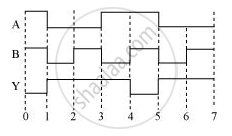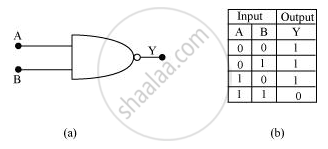Department of Pre-University Education, KarnatakaPUC Karnataka Science Class 12

# The Following Figure Shows the Input Waveforms (A, B) and the Output Waveform (Y) of a Gate. Identify the Gate, Write Its Truth Table and Draw Its Logic Symbol. - Physics

#### Question

The following figure shows the input waveforms (A, B) and the output waveform (Y) of a gate. Identify the gate, write its truth table and draw its logic symbol.#### Solution

The gate is the NAND gate.Is there an error in this question or solution?

#### Video TutorialsVIEW ALL 

The Following Figure Shows the Input Waveforms (A, B) and the Output Waveform (Y) of a Gate. Identify the Gate, Write Its Truth Table and Draw Its Logic Symbol. Concept: Digital Electronics and Logic Gates.